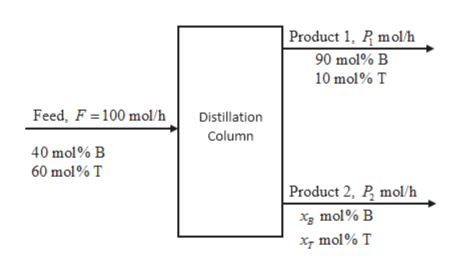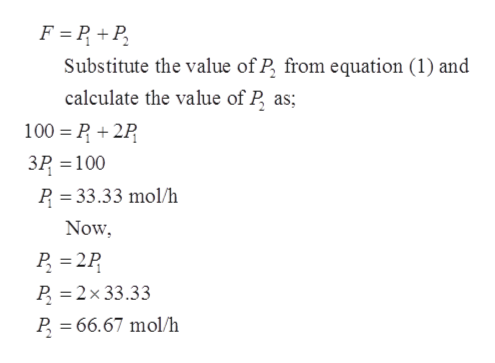# the feed to distillation column is 100gmole/h. the feed composition is 40 mol℅ benzene and the rest is toluene. there are two products P1 and P2. the ratio of the two products is 1/2 and P1 is 90 mol℅ benzene. Calculate the flow rate and composition of P2

Question
6 views
• the feed to distillation column is 100gmole/h. the feed composition is 40 mol℅ benzene and the rest is toluene. there are two products P1 and P2. the ratio of the two products is 1/2 and P1 is 90 mol℅ benzene. Calculate the flow rate and composition of P2
check_circle

Step 1

Let, Benzene = B and Toluene = T

xF = 0.4 mol B/mol (mole fraction of benzene in feed stream)

yB = 0.9 mol B/mol (mole fraction of benzene in product 1 stream)

Basis of the calculation is taken as 100 mol/h of feed. Schematic diagram of the given process is:help_outlineImage TranscriptioncloseProduct 1, P molh 90 mol% B 10 mol% T Feed, F 100 mol/h Distillation Column 40 mol% B 60 mol% T Product 2, P mol/h x mol% B xmol% T fullscreen
Step 2

The ratio of the two products is given as:

Step 3

Apply mole balance for the ...help_outlineImage TranscriptioncloseF P+P Substitute the value of P from equation (1) and calculate the value of P as 100 P2P 3P 100 P 33.33 mol/h = Now P 2P P 2x 33.33 P 66.67 mol/h fullscreen

### Want to see the full answer?

See Solution

#### Want to see this answer and more?

Solutions are written by subject experts who are available 24/7. Questions are typically answered within 1 hour.*

See Solution
*Response times may vary by subject and question.
Tagged in

### Chemical Technology